## Indicators

•Cited by SciELO
•Access statistics

## Related links

•Cited by Google
•Similars in SciELO
•Similars in Google

## Print version ISSN 0120-5609

### Ing. Investig. vol.31 no.3 Bogotá Sept./Dec. 2011

R-chaosoptimiser: an optimiser for unconstrained global nonlinear optimisation written in R language for statistical computing

R-chaosoptimiser: un optimizador global no lineal sin restricciones escrito en lenguaje R para el calculo estadístico

Juan David Velásquez H.1

1 PhD. in Engineering, Energy Systems Area, Universidad Nacional de Colombia, Medellín, Colombia (2009); Masters in Systems Engineering , Universidad Nacional de Colombia, Medellín, Colombia (1997); Associate professor, Escuela de Sistemas, Facultad de Minas, Universidad Nacional de Colombia.. Director of the Applied Computing Research Group, Facultad de Minas, Universidad Nacional de Colombia. jdvelasq@ unal.edu.co.

ABSTRACT

This paper discusses using R-chaosoptimiser, an R language package for nonlinear optimisation based on gradient techniques and chaos optimisation algorithms. Its implementation was based on three building blocks which could be executed alone or un combination: the first carrier wave algorithm, the chaos-based cyclical coordinate search method and the second wave carrier algorithm. Using chaos optimisation algorithms allows the tool to break away from local optimal points and converge towards an overall optimum inside a predefined search domain. Within the previous components, a user would be specifying the BFGS algorithm for refining the current best solution. Using the BFGS algorithm is not mandatory, so that its implementation was able to optimise problems having objective function discontinuities. However, the BFGS algorithm is a powerful local search method, meaning that it is used to exploit current knowledge about an objective function for improving a current solution; an explanatory example is presented.

Keywords: optimisation, R language, gradient-based method, chaos, algorithm.

RESUMEN

En este artículo se discute la implementación de rchaosoptimizer, un paquete de R para la optimización no lineal basada en técnicas de gradiente y algoritmos de optimización caóticos. La implementación está basada en tres bloques constructivos que pueden ser ejecutados solos o combinados: 1) el algoritmo de primera onda; 2) el método de búsqueda por coordenadas cíclicas basado en caos; y, 3) el algoritmo de segunda onda. El uso de algoritmos de optimización caóticos permite a la herramienta implementada escapar de puntos óptimos locales y converger al óptimo global dentro del domino predefinido de búsqueda. Dentro de los componentes previos, el usuario podría especificar una llamada al algoritmo BFGS para refinar la solución actual. El uso del algoritmo BFGS no es obligatorio, tal que la implementación es capaz de optimizar problemas con discontinuidades en la función objetivo. Sin embargo, el algoritmo BFGS es un método poderoso de búsqueda local, tal que, él es usado para explotar el conocimiento sobre la función objetivo para mejorar la solución actual. Finalmente, en ejemplo exploratorio es presentado.

Palabras clave: optimización, Lenguaje R, métodos basados en gradiente, caos, algoritmos.

Received: January 25th 2010 Accepted: October 6th 2011

Introduction

Solving nonlinear optimisation complex problems represents an important practical and research field. Developing algorithms and practical implementation have led to applications in mathematics, engineering, economics and computer sciences. Optimisation is usually difficult due to (Pardalos and Resende, 2002):

– Objective function complexity: analytical calculation of deri-vates is difficult if not impossible; moreover, even though derivates may be available, there are no mathematical formulas for estimating the overall optimum;

– Restrictions imposed on the problem: these are related to a feasible solution's complexity. Optimisation methods may be able to search inside such region and to discard unfeasible solutions;

– The presence of so-called multiple local minima: this is related to the complexity of the surface generated by the objective function, so that it is very difficult to evade local optimal points; and

– The limitations of many optimisation methodologies: or, in other words, how the methods are affected by the previous aspects and in which particular conditions a specific method is able to find good solutions within the search space.

The development, testing and implementation of heuristic-based optimisation algorithms is an important research field, especially for those based on stochastic optimisation (Pardalos and Re-sende, 2002). Well-known examples would include classical paradigms such as simulated annealing (Kirkpatrick, Gelatt and Vecchi, 1983), random search (Matyas, 1965; Solis and Wets, 1981) and genetic algorithms (Goldberg, 1989). However, new methodologies have emerged such as artificial immune systems (Farmer, Packard and Perelson, 1986; Bersiniand Varela, 1991), the fast clonal algorithm (Khilwani, Prakash and Shankar, 2008) and harmony search (Lee and Geem, 2005).

New optimisation methodologies based on using numerical sequences generated by means of a chaotic map (Li and Jiang, 1997; Choi and Lee, 1998; Tavazoei and Haeri, 2007; Yang, Li and Cheng, 2007; Velásquez, 2010) have emerged recently instead of random number generators. They are called chaos optimisation algorithms (COA).

This paper was aimed at introducing the R package R-chaosoptimiser, which is a programme for solving nonlinear optimisation problems stated as ƒ(x) subject to L ≤ x U , where x, L, U are vectors of n x 1, and ƒ Q is a nonlinear function, so that ƒ: Ra → R. Our programme consisted of four building blocks:

1. The first wave carrier or basic chaos optimising algorithm (Li and Jiang (1997);

2. The enhanced cyclical coordinate search method (Velásquez, 2010);

3. Li and Jiang's second wave carrier algorithm (1997); and

4. The BFGS gradient-based optimisation algorithm, which is used within any of the previous blocks for enhancing the current best solution (Yang, Li and Cheng, 2007; Velásquez, 2010).

Using chaos optimisation algorithms (Blocks 1, 2 and 3) allows a tool to avoid local optimal points and converge towards an overall optimum within a predefined search domain. Using the BFGS algorithm is not mandatory, so that its implementation was able to optimise problems involving objective function discontinuities. However, the BFGS algorithm is a powerful local search method, meaning that it is used to exploit current knowledge about an objective function, thereby improving a current solution.

Velásquez (2011) has analysed basic chaos optimising algorithm and first and second wave carrier patterns, with the optional use of gradient-based techniques, when four well-known nonlinear benchmark functions were optimised. The author concluded that the first wave carrier was unnecessary and the successful algorithm was due to a combination of second wave carrier methodology and gradient-based optimisation.

Velásquez (2010) has presented a new chaos optimisation algorithm for which the sampling mechanism was based on coordinate search methods and classical chaos optimisation algorithms; a gradient-based technique was used for refining the final solution. Evidence reported by Velásquez (2010) has indicated that the methodology so proposed was a strong alternative to other heuristic methods.

Chaotic maps

Chaos is understood to be complex, bounded and unstable behaviour caused by a simple deterministic nonlinear system or chaotic map so that generated sequences are quasi-random, irregular, ergodic, semi-stochastic and very sensitive to an initial value (Strogatz, 2000). Using chaotic sequences instead of quasi-random number generators seems to be a powerful strategy for improving many traditional heuristic algorithms and their main use is to avoid local minima points (Caponetto, Fortuna, Fazzino and Xibilia, 2003). The following chaotic maps were used in the R-chaosoptimiser programme:

1. A logistic map: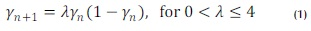2. A new chaotic map: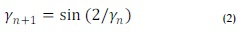3. A sine map: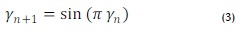4. A tent map: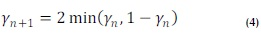Algorithms used in this work

This section describes optimisation algorithms used in the R-chaosoptimiser programme.

First carrier wave (fcw) algorithm

The so-called first carrier wave algorithm is the most elementary procedure for generating candidate points xc inside a feasible region; optimum, Xi, is the candidate point having the lowest value for ƒ (xc). The process is schematised in Figure 1. Candidate points Xc (line 05) were generated in domain [L, U] by means of chaotic sequence vector y; each component of y, y(i) was thus mapped linearly to interval [L(i), U(i)]. It has been assumed in Figure 1 that the components of y were restricted to interval [0, 1] as occurs for the logistic map. A new chaotic sequence vector was generated at each iteration using chaotic map H 0 (line 12); for example, each component was generated using y(i) = 4y(i) [1-y(i)] , if H () were the logistic map. The current local optimum x1 was saved in line 09. In addition, every ƒ CW.C2 iterations the current local optimum would be refined by means of a gradient-based optimisation algorithm (line 14). In this case, g() was the implementation of the BFGS algorithm in R language optim function. The complete process was repeated few. Ci times (from line 02 to line 15).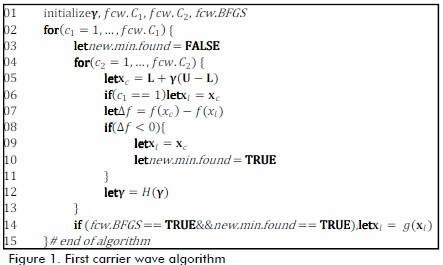Enhanced cyclical coordinate search (CCS) algorithm

Objective function ƒ (x) was minimised along a unitary vector coinciding with one of the coordinates axes in the enhanced cyclical coordinate search algorithm (Figure 2) proposed by Velásquez (2010). A new candidate point was obtained from the current best solution (line 08) by changing the i-th component for a random value inside the interval centred on the current best value (for the i-th component) having radius r (line 09). >This sampling was repeated ccs.K2 times (line 06), each time obtaining a better point and thus updating the best current solution (from lines 11 to 13). The complete cycle was repeated ccs.K1 times (line 02).

Each time a cycle was completed on the whole axis, the current best solution was optionally refined using the BFGS gradient-based optimisation algorithm (line 17). The following methods were provided for calculating r:

Second carrier wave (scw) algorithm

The second wave carrier algorithm (Figure 3) consisted of a local search around X1. γ is a chaotic sequence vector and r is a scalar parameter related to the search radius around X1. r is calculated in each iteration by means of function QO (line 04). Each candidate point was generated inside hypercube [xl - r, xl + r], since each component of y (with domain [0, 1]) was mapped to interval [-r, r]. The local optima was updated each time a better point was found (lines 08 to 11) so that the procedure continued around the new optimum. The search procedure was similar to the simulated annealing technique where ascending movements were not allowed. The following methods were provided for calculating r :

Example

An example of using the R-chaosoptimiser programme with Rosenbrock function minimisation is given below: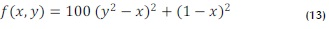This has been plotted in Figure 4. The above function would be generalised to n-dimensions by means of the following transformation: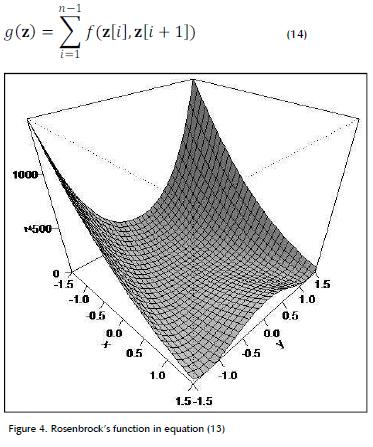A function was first created for optimising (14):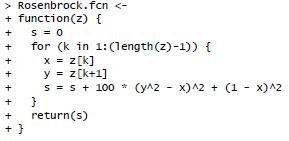The second carrier wave algorithm was just applied for optimising this function; R-chaosoptimiser was then used using this function: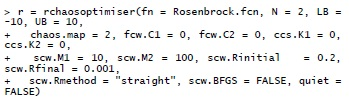The first argument in this example was the function to be optimised, the second argument was the number of objective function dimensions, the third and fourth arguments were the lower and upper limits for independent variables, the fifth argument was the logistic map and the remaining arguments were the values for the constants described in the algorithms shown in Figures 1, 2 and 3. The last argument produced a verbose output for the current run.

The output produced by the above was as follows:
11/25/09 14:34:18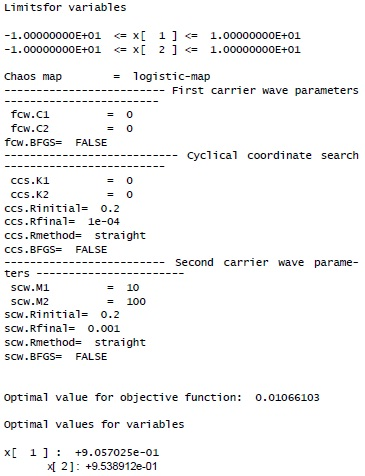The printed output consisted of the following blocks: run date and time, the variables' limits, the chaos map used, the algorithm's parameters' values, the objective function optimal value and the variables' optimal values.

The search procedure was refined by using the BFGS algorithm inside the second carrier wave algorithm, as follows: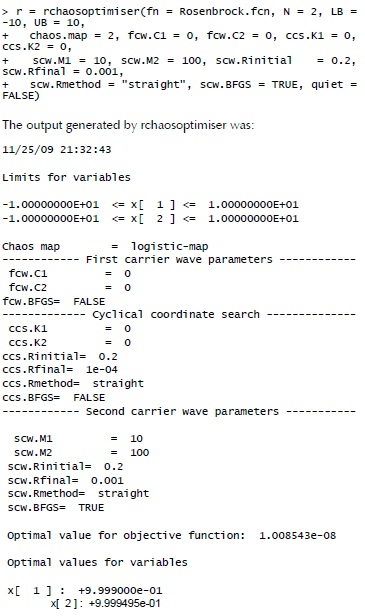Conclusions

This paper has discussed R-chaosoptimiser, an R language package for nonlinear optimisation based on gradient techniques and chaos optimisation algorithms. Our implementation was based on three building blocks which could be executed alone or combined: the first carrier wave algorithm, the chaos-based cyclical coordinate search method and the second wave carrier algorithm. Chaos algorithms are used for defining solution space sampling mechanism and allow an algorithm to avoid using local optimal points. Gradient-based techniques were used to refine the current solution. A user would specify the BFGS algorithm for refining the current best solution inside the previous building blocks. Using the BFGS algorithm is not mandatory, so that our implementation was able to optimise problems involving discontinuities in the objective function.

Bibliography

Bersini, H., Varela, F.J.,Hints for adaptive problem solving gleaned from immune networks., Lecture Notes in Computer Science, Vol. 496, 1991, pp. 343-354.        [ Links ]

Caponetto, R., Fortuna, L.,Fazzino, S., Xibilia, M. G., Chaotic sequences to improve the performance of evolutionary algorithms., IEEE Transactions on Evolutionary Computation, Vol. 7, No. 3, 2003, pp. 289-304.        [ Links ]

Choi, C., Lee, J.J., Chaotic local search algorithm., Artificial Life and Robotics, Vol. 2, No. 1, 1998, pp. 41-47.        [ Links ]

Farmer, J.D., Packard, N., Perelson, A., The immune system, adaptation and machine learning.,Physica D, Vol. 2, 1986, pp. 187-204.        [ Links ]

Lee, K.S., Geem, Z.W., A new meta-heuristic algorithm for continuous engineering optimization: harmony search theory and practice., Computer Methods in Applied Mechanics and Engineering, Vol. 194, No. 36-38, 2005, pp. 3902-3933.        [ Links ]

Li, B., Jiang, W.-S., Chaos optimization method and its application., Control Theory and Application, Vol. 14, No. 4, 1997, pp. 613-615.        [ Links ]

Goldberg, D. E., Genetic Algorithms in Search, Optimization and Machine Learning., Boston, MA, Kluwer Academic Publishers, 1989.        [ Links ]

Khilwani, N.,Prakash, A., Shankar, R.,Tiwari, M.K., Fast clonal algorithm., Engineering Applications of Artificial Intelligence, Vol. 21, No. 1, 2008, pp. 106-128.        [ Links ]

Kirkpatrick, S.,Gelatt, C. D.,Vecchi, M. P., Optimization by simulated annealing., Science, Vol. 220, No. 4598, 1983, pp. 671-680.        [ Links ]

Matyas, J., Random optimization., Automation and Remote Control, Vol. 26, 1965, pp. 246-253.        [ Links ]

Pardalos, P. M.,Resende, M. G. C.,(ed.),Handbook of Applied Optimization., New York, Oxford University Press, 2002.        [ Links ]

Solis, F.J., Wets, J.B., Minimization by random search techniques., Mathematics of Operations Research, Vol. 6, No. 1, 1981, pp. 19-30.        [ Links ]

Strogatz, S. H., Nonlinear dynamics and chaos., Massachussetts, Perseus Publishing, 2000.        [ Links ]

Tavazoei, M. S.,Haeri, M., An optimization algorithm based on chaotic behavior and fractal nature., Journal of Computational and Applied Mathematics, Vol. 206, No. 2, 2007, pp. 1070-1081.        [ Links ]

Velásquez, J. D., An Enhanced Hybrid Chaotic Algorithmusing Cyclic Coordinate Search and Gradient Techniques., Revista de Ingeniería, Vol. 32, 2010, pp. 45-53.        [ Links ]

Velásquez, J. D., Una introducción a los algoritmos basados en caos para optimización numérica., Revista Avances en Sistemas e Informática, Vol. 8, No. 1, 2011, pp. 51-60.        [ Links ]

Yang, D., Li, G., Cheng, G., On the efficiency of chaos optimization algorithms for global optimization., Chaos, Solitons and Fractals, Vol. 34, 2007, pp. 1366-1375.        [ Links ]All the contents of this journal, except where otherwise noted, is licensed under a Creative Commons Attribution License